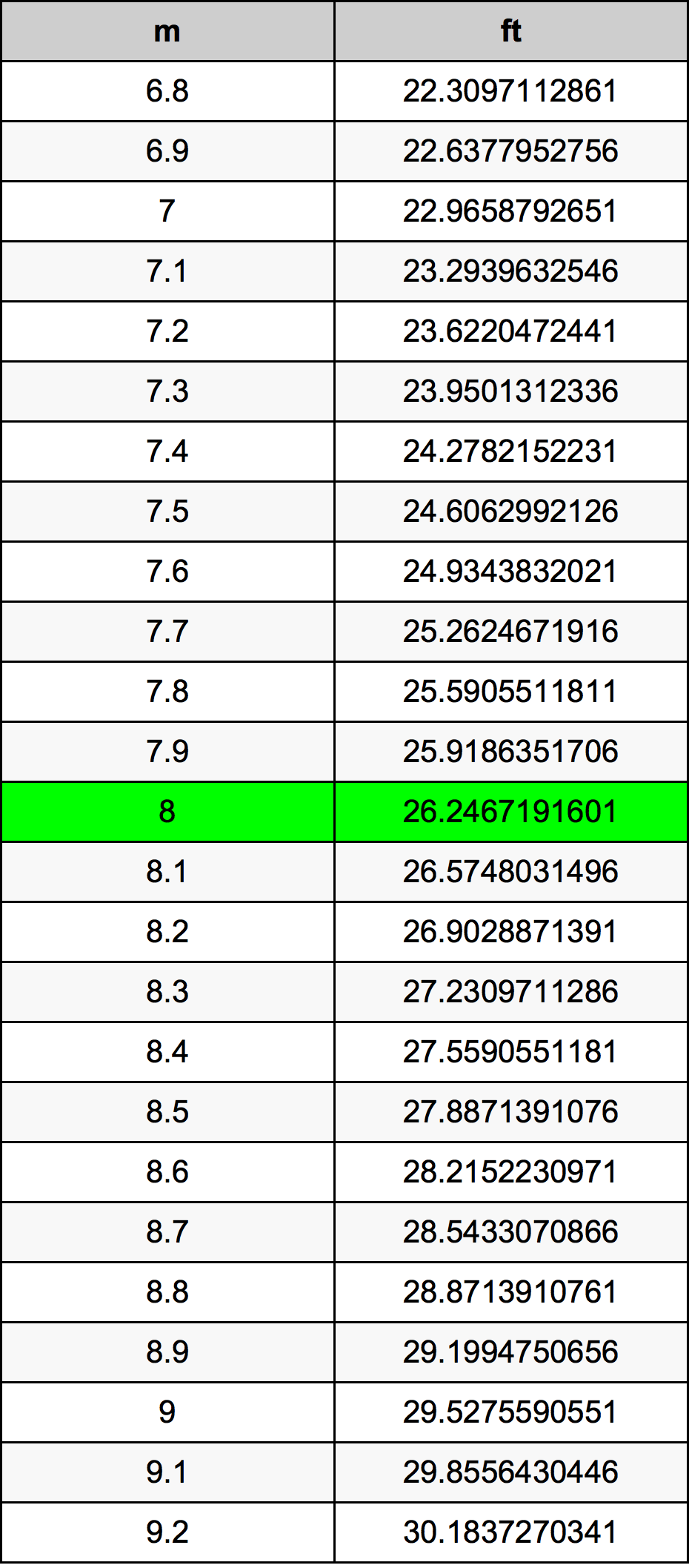Meters To Feet

# 8 m to ft8 Meters to Feet

m
=
ft

## How to convert 8 meters to feet?

 8 m * 3.280839895 ft = 26.2467191601 ft 1 m
A common question isHow many meter in 8 foot?And the answer is 2.4384 m in 8 ft. Likewise the question how many foot in 8 meter has the answer of 26.2467191601 ft in 8 m.

## How much are 8 meters in feet?

8 meters equal 26.2467191601 feet (8m = 26.2467191601ft). Converting 8 m to ft is easy. Simply use our calculator above, or apply the formula to change the weight 8 m to ft.

## Convert 8 m to common lengths

UnitLength
Nanometer8000000000.0 nm
Micrometer8000000.0 µm
Millimeter8000.0 mm
Centimeter800.0 cm
Inch314.960629921 in
Foot26.2467191601 ft
Yard8.7489063867 yd
Meter8.0 m
Kilometer0.008 km
Mile0.0049709695 mi
Nautical mile0.0043196544 nmi

## 8 Meter Conversion Table## Alternative spelling

8 Meter to Feet, 8 Meter in Feet, 8 Meters to Foot, 8 Meters in Foot, 8 m to Foot, 8 m in Foot, 8 Meter to ft, 8 Meter in ft, 8 Meter to Foot, 8 Meter in Foot, 8 Meters to ft, 8 Meters in ft, 8 Meters to Feet, 8 Meters in Feet